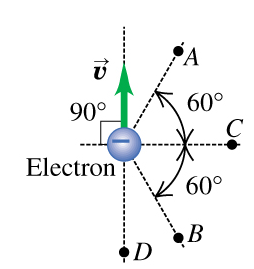# Problem: An electron moves at 0.150 c as shown in the figure (Figure 1). There are points: A, B, C, and D 2.10 μm from the electron. Find the magnitude of the magnetic field this electron produces at the pointno title providedA) Find the magnitude and direction of the magnetic field this electron produces at the point A.B) Find the magnitude and direction of the magnetic field this electron produces at the point B.C) Find the magnitude and direction of the magnetic field this electron produces at the point C.D) Find the magnitude and direction of the magnetic field this electron produces at the point D.

###### FREE Expert Solution

The magnetic field is expressed as:

$\overline{){\mathbf{B}}{\mathbf{=}}\frac{{\mathbf{\mu }}_{\mathbf{0}}}{\mathbf{4}\mathbf{\pi }}\frac{\mathbf{q}\mathbf{\left(}\stackrel{\mathbf{⇀}}{\mathbf{v}}\mathbf{×}\stackrel{\mathbf{^}}{\mathbf{r}}}{{\mathbf{r}}^{\mathbf{2}}}}$

The magnitude of the magnetic field is then:

$\mathbit{B}\mathbf{=}\frac{{\mathbf{\mu }}_{\mathbf{0}}\mathbf{q}\mathbf{v}\mathbf{s}\mathbf{i}\mathbf{n}\mathbf{\theta }}{\mathbf{4}\mathbf{\pi }{\mathbf{r}}^{\mathbf{2}}}$

But, $\frac{{\mathbf{\mu }}_{\mathbf{0}}\mathbf{q}\mathbf{v}}{\mathbf{4}\mathbf{\pi }{\mathbf{r}}^{\mathbf{2}}}{\mathbf{=}}{{\mathbit{B}}}_{{\mathbf{0}}}$

Therefore,

$\overline{){\mathbf{B}}{\mathbf{=}}{{\mathbf{B}}}_{{\mathbf{0}}}{\mathbf{s}}{\mathbf{i}}{\mathbf{n}}{\mathbf{\theta }}}$

We'll also use the left-hand rule to determine the direction of the magnetic field.

This is because the electron is negatively charged and we use the left-hand rule for negative charges.

91% (303 ratings)###### Problem Details

An electron moves at 0.150 c as shown in the figure (Figure 1). There are points: A, B, C, and D 2.10 μm from the electron. Find the magnitude of the magnetic field this electron produces at the pointno title provided

A) Find the magnitude and direction of the magnetic field this electron produces at the point A.

B) Find the magnitude and direction of the magnetic field this electron produces at the point B.

C) Find the magnitude and direction of the magnetic field this electron produces at the point C.

D) Find the magnitude and direction of the magnetic field this electron produces at the point D.

Frequently Asked Questions

What scientific concept do you need to know in order to solve this problem?

Our tutors have indicated that to solve this problem you will need to apply the Magnetic Field Produced by Moving Charges concept. You can view video lessons to learn Magnetic Field Produced by Moving Charges. Or if you need more Magnetic Field Produced by Moving Charges practice, you can also practice Magnetic Field Produced by Moving Charges practice problems.